# pdeplot3D

Plot solution or surface mesh for 3-D problem

## Syntax

``pdeplot3D(results.Mesh,ColorMapData=results.NodalSolution)``
``pdeplot3D(results.Mesh,ColorMapData=results.Temperature)``
``pdeplot3D(results.Mesh,ColorMapData=results.VonMisesStress,Deformation=results.Displacement)``
``pdeplot3D(results.Mesh,ColorMapData=results.ElectricPotential)``
``pdeplot3D(mesh)``
``pdeplot3D(nodes,elements)``
``pdeplot3D(model)``
``pdeplot3D(___,Name,Value)``
``h = pdeplot3D(___)``

## Description

example

````pdeplot3D(results.Mesh,ColorMapData=results.NodalSolution)` plots the solution at nodal locations.```

example

````pdeplot3D(results.Mesh,ColorMapData=results.Temperature)` plots the temperature at nodal locations for a 3-D thermal analysis problem.```

example

````pdeplot3D(results.Mesh,ColorMapData=results.VonMisesStress,Deformation=results.Displacement)` plots the von Mises stress and shows the deformed shape for a 3-D structural analysis problem.```

example

````pdeplot3D(results.Mesh,ColorMapData=results.ElectricPotential)` plots the electric potential at nodal locations for a 3-D electrostatic analysis problem.```

example

````pdeplot3D(mesh)` plots the mesh.```

example

````pdeplot3D(nodes,elements)` plots the mesh defined by `nodes` and `elements`.```

example

````pdeplot3D(model)` plots the surface mesh specified in `model`. This syntax does not work with an `femodel` object.```

example

````pdeplot3D(___,Name,Value)` plots the surface mesh, the data at nodal locations, or both the mesh and data, depending on the `Name,Value` pair arguments. Use any arguments from the previous syntaxes.```
````h = pdeplot3D(___)` returns a handle to a plot, using any of the previous syntaxes.```

## Examples

collapse all

Plot a PDE solution on the geometry surface. First, create a PDE model and import a 3-D geometry file. Specify boundary conditions and coefficients. Mesh the geometry and solve the problem.

```model = createpde; importGeometry(model,"Block.stl"); applyBoundaryCondition(model,"dirichlet",Face=1:4,u=0); specifyCoefficients(model,m=0,d=0,c=1,a=0,f=2); generateMesh(model); results = solvepde(model)```
```results = StationaryResults with properties: NodalSolution: [12691x1 double] XGradients: [12691x1 double] YGradients: [12691x1 double] ZGradients: [12691x1 double] Mesh: [1x1 FEMesh] ```

Plot the solution at the nodal locations on the geometry surface.

```u = results.NodalSolution; msh = results.Mesh; pdeplot3D(model,ColorMapData=u)```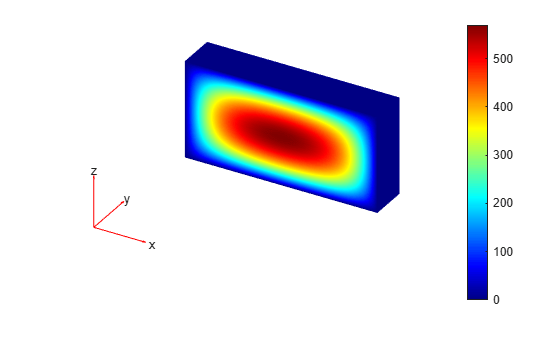Solve a 3-D steady-state thermal problem.

Create a thermal model for this problem.

`thermalmodel = createpde(thermal="steadystate");`

Import and plot the block geometry.

```importGeometry(thermalmodel,"Block.stl"); pdegplot(thermalmodel,FaceLabels="on",FaceAlpha=0.5) axis equal```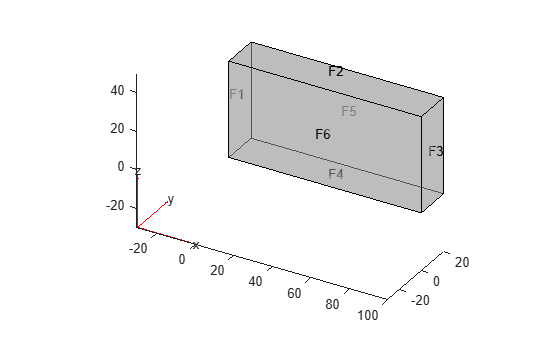Assign material properties.

`thermalProperties(thermalmodel,ThermalConductivity=80);`

Apply a constant temperature of 100 °C to the left side of the block (face 1) and a constant temperature of 300 °C to the right side of the block (face 3). All other faces are insulated by default.

```thermalBC(thermalmodel,Face=1,Temperature=100); thermalBC(thermalmodel,Face=3,Temperature=300);```

Mesh the geometry and solve the problem.

```generateMesh(thermalmodel); thermalresults = solve(thermalmodel)```
```thermalresults = SteadyStateThermalResults with properties: Temperature: [12691x1 double] XGradients: [12691x1 double] YGradients: [12691x1 double] ZGradients: [12691x1 double] Mesh: [1x1 FEMesh] ```

The solver finds the temperatures and temperature gradients at the nodal locations. To access these values, use `thermalresults.Temperature`, `thermalresults.XGradients`, and so on. For example, plot temperatures at the nodal locations.

`pdeplot3D(thermalresults.Mesh,ColorMapData=thermalresults.Temperature)`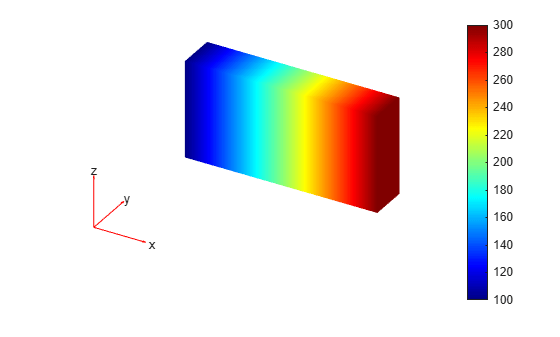For a 3-D steady-state thermal model, evaluate heat flux at the nodal locations and at the points specified by `x`, `y`, and `z` coordinates.

Create a thermal model for steady-state analysis.

`thermalmodel = createpde(thermal="steadystate");`

Create the following 3-D geometry and include it in the model.

```importGeometry(thermalmodel,"Block.stl"); pdegplot(thermalmodel,FaceLabels="on",FaceAlpha=0.5) title("Copper block, cm") axis equal```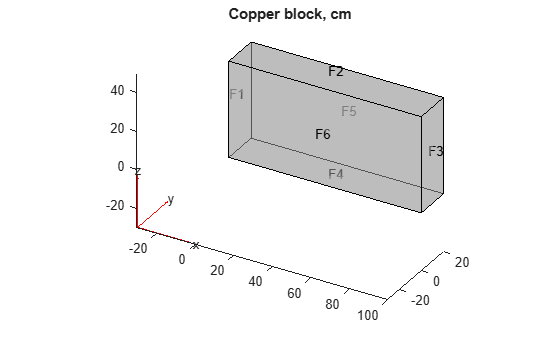Assuming that this is a copper block, the thermal conductivity of the block is approximately $4\phantom{\rule{0.16666666666666666em}{0ex}}W/\left(cmK\right)$.

`thermalProperties(thermalmodel,ThermalConductivity=4);`

Apply a constant temperature of 373 K to the left side of the block (face 1) and a constant temperature of 573 K to the right side of the block (face 3).

```thermalBC(thermalmodel,Face=1,Temperature=373); thermalBC(thermalmodel,Face=3,Temperature=573);```

Apply a heat flux boundary condition to the bottom of the block.

`thermalBC(thermalmodel,Face=4,HeatFlux=-20);`

Mesh the geometry and solve the problem.

```generateMesh(thermalmodel); thermalresults = solve(thermalmodel)```
```thermalresults = SteadyStateThermalResults with properties: Temperature: [12691x1 double] XGradients: [12691x1 double] YGradients: [12691x1 double] ZGradients: [12691x1 double] Mesh: [1x1 FEMesh] ```

Evaluate heat flux at the nodal locations.

```[qx,qy,qz] = evaluateHeatFlux(thermalresults); figure pdeplot3D(thermalresults.Mesh,FlowData=[qx qy qz])```Create a grid specified by `x`, `y`, and `z` coordinates, and evaluate heat flux to the grid.

```[X,Y,Z] = meshgrid(1:26:100,1:6:20,1:11:50); [qx,qy,qz] = evaluateHeatFlux(thermalresults,X,Y,Z);```

Reshape the `qx`, `qy`, and `qz` vectors, and plot the resulting heat flux.

```qx = reshape(qx,size(X)); qy = reshape(qy,size(Y)); qz = reshape(qz,size(Z)); figure quiver3(X,Y,Z,qx,qy,qz)```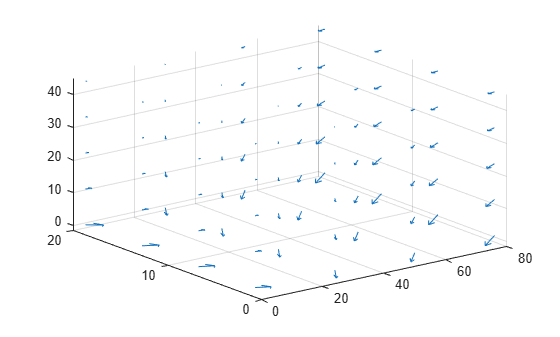Alternatively, you can specify the grid by using a matrix of query points.

```querypoints = [X(:) Y(:) Z(:)]'; [qx,qy,qz] = evaluateHeatFlux(thermalresults,querypoints); qx = reshape(qx,size(X)); qy = reshape(qy,size(Y)); qz = reshape(qz,size(Z)); figure quiver3(X,Y,Z,qx,qy,qz)```Create a structural analysis model for a 3-D problem.

`structuralmodel = createpde(structural="static-solid");`

Import the geometry and plot it.

```importGeometry(structuralmodel,"SquareBeam.stl"); pdegplot(structuralmodel,FaceLabels="on",FaceAlpha=0.5)```Specify Young's modulus and Poisson's ratio.

```structuralProperties(structuralmodel,PoissonsRatio=0.3, ... YoungsModulus=210E3);```

Specify that face 6 is a fixed boundary.

`structuralBC(structuralmodel,Face=6,Constraint="fixed");`

Specify the surface traction for face 5.

```structuralBoundaryLoad(structuralmodel,Face=5, ... SurfaceTraction=[0;0;-2]);```

Generate a mesh and solve the problem.

```generateMesh(structuralmodel); structuralresults = solve(structuralmodel);```

Plot the deformed shape with the von Mises stress using the default scale factor. By default, `pdeplot3D` internally determines the scale factor based on the dimensions of the geometry and the magnitude of deformation.

```figure pdeplot3D(structuralresults.Mesh, ... ColorMapData=structuralresults.VonMisesStress, ... Deformation=structuralresults.Displacement)```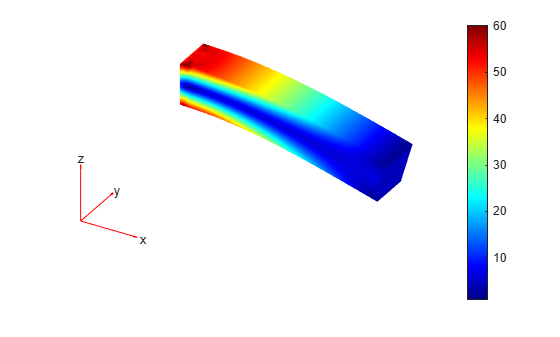Plot the same results with the scale factor 500.

```figure pdeplot3D(structuralresults.Mesh, ... ColorMapData=structuralresults.VonMisesStress, ... Deformation=structuralresults.Displacement, ... DeformationScaleFactor=500)```Plot the same results without scaling.

```figure pdeplot3D(structuralresults.Mesh, ... ColorMapData=structuralresults.VonMisesStress)```Evaluate the von Mises stress in a beam under a harmonic excitation.

Create a transient dynamic model for a 3-D problem.

`structuralmodel = createpde(structural="transient-solid");`

Create the geometry and include it in the model. Plot the geometry.

```gm = multicuboid(0.06,0.005,0.01); structuralmodel.Geometry = gm; pdegplot(structuralmodel,FaceLabels="on",FaceAlpha=0.5) view(50,20)```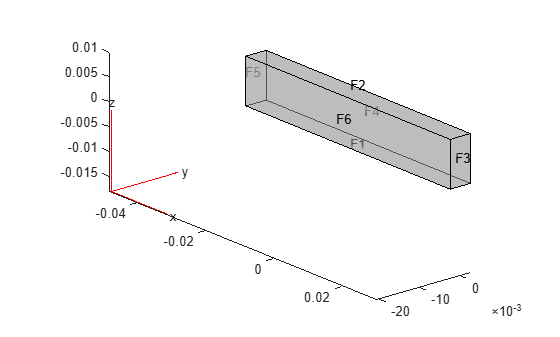Specify Young's modulus, Poisson's ratio, and the mass density of the material.

```structuralProperties(structuralmodel,YoungsModulus=210E9, ... PoissonsRatio=0.3, ... MassDensity=7800);```

Fix one end of the beam.

`structuralBC(structuralmodel,Face=5,Constraint="fixed");`

Apply a sinusoidal displacement along the y-direction on the end opposite the fixed end of the beam.

```structuralBC(structuralmodel,Face=3, ... YDisplacement=1E-4, ... Frequency=50);```

Generate a mesh.

`generateMesh(structuralmodel,Hmax=0.01);`

Specify the zero initial displacement and velocity.

`structuralIC(structuralmodel,Displacement=[0;0;0],Velocity=[0;0;0]);`

Solve the model.

```tlist = 0:0.002:0.2; structuralresults = solve(structuralmodel,tlist);```

Evaluate the von Mises stress in the beam.

`vmStress = evaluateVonMisesStress(structuralresults);`

Plot the von Mises stress for the last time-step.

```figure pdeplot3D(structuralresults.Mesh,ColorMapData = vmStress(:,end)) title("von Mises Stress in the Beam for the Last Time-Step")```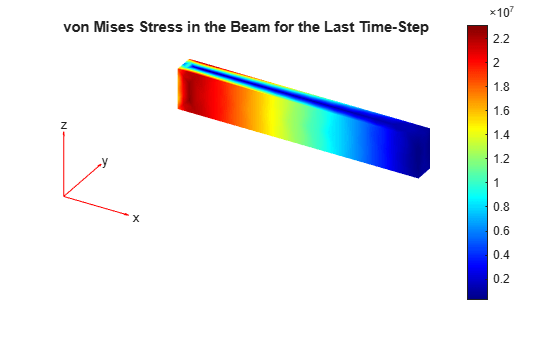Solve an electromagnetic problem and find the electric potential and field distribution for a 3-D geometry representing a plate with a hole.

Create an electromagnetic model for electrostatic analysis.

`emagmodel = createpde(electromagnetic="electrostatic");`

Import and plot the geometry representing a plate with a hole.

```gm = importGeometry(emagmodel,"PlateHoleSolid.stl"); pdegplot(gm,FaceLabels="on",FaceAlpha=0.3)```Specify the vacuum permittivity in the SI system of units.

`emagmodel.VacuumPermittivity = 8.8541878128E-12;`

Specify the relative permittivity of the material.

`electromagneticProperties(emagmodel,RelativePermittivity=1);`

Specify the charge density for the entire geometry.

`electromagneticSource(emagmodel,ChargeDensity=5E-9);`

Apply the voltage boundary conditions on the side faces and the face bordering the hole.

```electromagneticBC(emagmodel,Voltage=0,Face=3:6); electromagneticBC(emagmodel,Voltage=1000,Face=7);```

Generate the mesh.

`generateMesh(emagmodel);`

Solve the model.

`R = solve(emagmodel)`
```R = ElectrostaticResults with properties: ElectricPotential: [4359x1 double] ElectricField: [1x1 FEStruct] ElectricFluxDensity: [1x1 FEStruct] Mesh: [1x1 FEMesh] ```

Plot the electric potential.

```figure pdeplot3D(R.Mesh,ColorMapData=R.ElectricPotential)```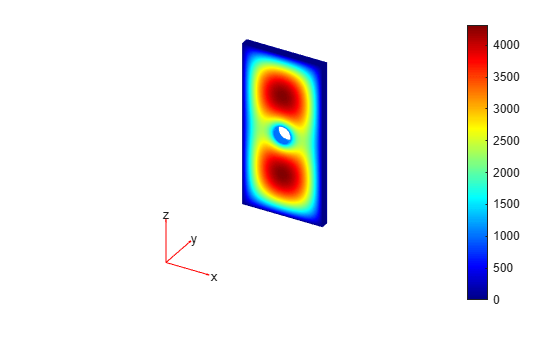Plot the electric field.

```pdeplot3D(R.Mesh,FlowData=[R.ElectricField.Ex ... R.ElectricField.Ey ... R.ElectricField.Ez])```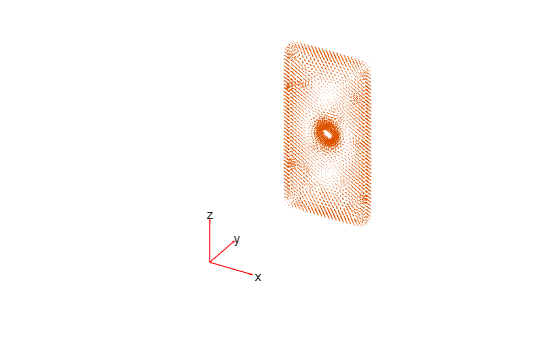Create a PDE model, include the geometry, and generate a mesh.

```model = createpde; importGeometry(model,"Tetrahedron.stl"); mesh = generateMesh(model,Hmax=20,GeometricOrder="linear");```

Plot the surface mesh.

`pdeplot3D(mesh)`Alternatively, you can plot a mesh by using `model` as an input argument.

`pdeplot3D(model)`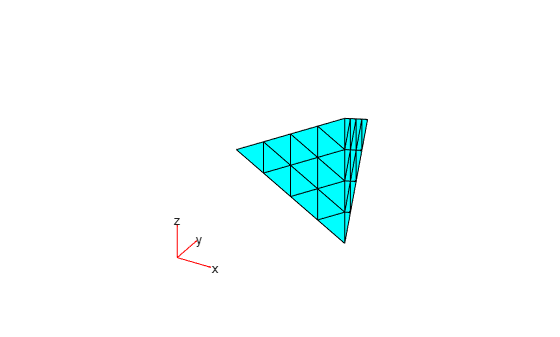Another approach is to use the nodes and elements of the mesh as input arguments for `pdeplot3D`.

`pdeplot3D(mesh.Nodes,mesh.Elements)`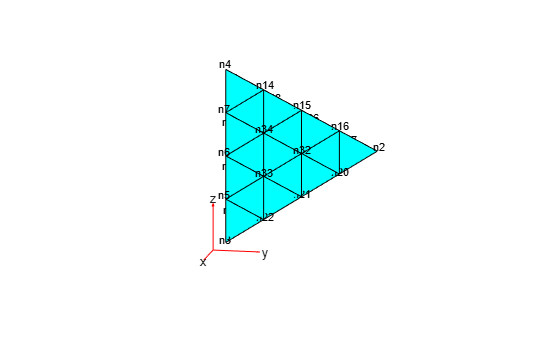Display the node labels on the surface of a simple mesh.

```pdeplot3D(mesh,NodeLabels="on") view(101,12)```Display the element labels.

```pdeplot3D(mesh,ElementLabels="on") view(101,12)```## Input Arguments

collapse all

Model container, specified as a `PDEModel` object, `ThermalModel` object, `StructuralModel` object, or `ElectromagneticModel` object.

Mesh description, specified as an `FEMesh` object. See FEMesh Properties.

Nodal coordinates, specified as a 3-by-NumNodes matrix. NumNodes is the number of nodes.

Element connectivity matrix in terms of the node IDs, specified as a 4-by-NumElements or 10-by-NumElements matrix. Linear meshes contain only corner nodes. For linear meshes, the connectivity matrix has four nodes per 3-D element. Quadratic meshes contain corner nodes and nodes in the middle of each edge of an element. For quadratic meshes, the connectivity matrix has 10 nodes per 3-D element.### Name-Value Arguments

Specify optional pairs of arguments as `Name1=Value1,...,NameN=ValueN`, where `Name` is the argument name and `Value` is the corresponding value. Name-value arguments must appear after other arguments, but the order of the pairs does not matter.

Before R2021a, use commas to separate each name and value, and enclose `Name` in quotes.

Example: `pdeplot3D(model,NodeLabels="on")`

Data to plot as a colored surface, specified as the comma-separated pair consisting of `"ColorMapData"` and a column vector with the number of elements that equals the number of points in the mesh. Typically, this data is the solution returned by `solvepde` for a scalar PDE problem and a component of the solution for a multicomponent PDE system.

Example: `ColorMapData=results.NodalSolution`

Example: `ColorMapData=results.NodalSolution(:,1)`

Data Types: `double`

Data for the quiver plot, specified as the comma-separated pair consisting of `"FlowData"` and an `M`-by-`3` matrix, where `M` is the number of mesh nodes. `FlowData` contains the x, y, and z values of the field at the mesh points. Set `FlowData` as follows:

```results = solvepde(model); [cgradx,cgrady,cgradz] = evaluateCGradient(results); pdeplot3D(results.Mesh,FlowData=[cgradx cgrady cgradz])```

`pdeplot3D` plots the real part of complex data.

Example: ```FlowData=[cgradx cgrady cgradz]```

Data Types: `double`

Indicator to show the mesh, specified as the comma-separated pair consisting of `"Mesh"` and `"on"` or `"off"`. Specify `"on"` to show the mesh in the plot.

Example: `Mesh="on"`

Data Types: `char` | `string`

Node labels, specified as the comma-separated pair consisting of `"NodeLabels"` and `"off"` or `"on"`.

Example: `NodeLabels="on"`

Data Types: `char` | `string`

Element labels, specified as the comma-separated pair consisting of `"ElementLabels"` and `"off"` or `"on"`.

Example: `ElementLabels="on"`

Data Types: `char` | `string`

Surface transparency for 3-D geometry, specified as a real number from `0` through `1`. The default value `1` indicates no transparency. The value `0` indicates complete transparency.

Example: `FaceAlpha=0.5`

Data Types: `double`

Deformed shape for structural analysis models, specified as the comma-separated pair consisting of `Deformation` and the `FEStruct` object representing displacement values at nodes. The displacement `FEStruct` object is a property of `StaticStructuralResults`, `TransientStructuralResults`, and `FrequencyStructuralResults`.

In an undeformed shape, center nodes in quadratic meshes are always added at half-distance between corners. When you plot a deformed shape, the center nodes might move away from the edge centers.

Example: `Deformation=results.Displacement`

Scaling factor for plotting the deformed shape, specified as the comma-separated pair consisting of `DeformationScaleFactor` and a positive number. Use this argument together with the `Deformation` name-value pair argument. The `pdeplot3D` function chooses the default value based on the geometry itself and on the magnitude of deformation.

Example: `DeformationScaleFactor=1000`

Data Types: `double`

## Output Arguments

collapse all

Handles to graphics objects, returned as a vector.

## Version History

Introduced in R2015a

expand all